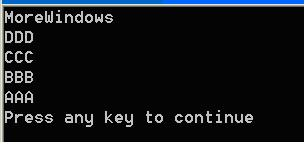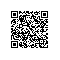# C++ 类的静态成员详细讲解

C++中，静态成员是属于整个类的而不是某个对象，静态成员变量只存储一份供所有对象共用。所以在所有对象中都可以共享它。使用静态成员变量实现多个对象之间的数据共享不会破坏隐藏的原则，保证了安全性还可以节省内存。

C++中类的静态成员变量和静态成员函数是个容易出错的地方，本文先通过几个例子来总结静态成员变量和成员函数使用规则，再给出一个实例来加深印象。希望阅读本文可以使读者对类的静态成员变量和成员函数有更为深刻的认识。

class Point

{

public:

void init()

{

}

static void output()

{

}

};

void main()

{

Point::init();

Point::output();

}

void main()

{

Point pt;

pt.init();

pt.output();

}

#include <stdio.h>

class Point

{

public:

void init()

{

}

static void output()

{

printf("%d\n", m_x);

}

private:

int m_x;

};

void main()

{

Point pt;

pt.output();

}

class Point

{

public:

void init()

{

output();

}

static void output()

{

}

};

void main()

{

Point pt;

pt.output();

}

#include <stdio.h>

class Point

{

public:

Point()

{

m_nPointCount++;

}

~Point()

{

m_nPointCount--;

}

static void output()

{

printf("%d\n", m_nPointCount);

}

private:

static int m_nPointCount;

};

void main()

{

Point pt;

pt.output();

}

Ctrl+F7编译无错误，按F7生成EXE程序时报链接错误

error LNK2001: unresolved external symbol "private: static int Point::m_nPointCount" (?m_nPointCount@Point@@0HA)

main()函数前加上int Point::m_nPointCount = 0;

#include <stdio.h>

#include <string.h>

const int MAX_NAME_SIZE = 30;

class Student

public:

Student(char *pszName);

~Student();

public:

static void PrintfAllStudents();

private:

char    m_name[MAX_NAME_SIZE];

Student *next;

Student *prev;

};

Student::Student(char *pszName)

strcpy(this->m_name, pszName);

//建立双向链表，新数据从链表头部插入。

this->prev = NULL;

Student::~Student ()//析构过程就是节点的脱离过程

{

}

else

{

this->prev->next = this->next;

this->next->prev = this->prev;

}

void Student::PrintfAllStudents()

{

for (Student *p = m_head; p != NULL; p = p->next)

printf("%s\n", p->m_name);

}

void main()

{

Student studentA("AAA");

Student studentB("BBB");

Student studentC("CCC");

Student studentD("DDD");

Student student("MoreWindows");

Student::PrintfAllStudents();

}使用钉钉扫一扫加入圈子
+ 订阅